# LOG#028. Rockets and relativity.The second post in this special thread of 3 devoted to Neil Armstrong memory has to do with rocketry.

Firstly, for completion, we are going to study the motion of a rocket in “vacuum” according to classical physics. Then, we will deduce the relatistic rocket equation and its main properties.

CLASSICAL NON-RELATIVISTIC ROCKETS

The fundamental law of Dynamics, following Sir Isaac Newton, reads: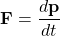Suppose a rocket with initial mass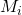and initial velocity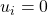. It ejects mass of propellant “gas” with “gas speed” (particles of gas have a relative velocity or speed with respect to the rest observer when the rocket move at speed) equals to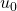(note that the relative speed will be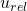and the propellant mass is. Generally, this speed is also called “exhaust velocity” by engineers. The motion of a variable mass or rocket is given by the so-called Metcherski’s equation:where. The Metcherski’s equation can be derived as follows: the rocket changes its mass and velocity so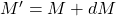and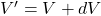, so the change in momentum is equal to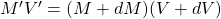, plus an additional term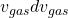and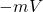. Therefore, the total change in momentum: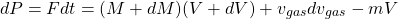Neglecting second order differentials, and setting the conservation of mass (we are in the non-relativistic case)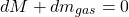we recover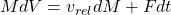that represents (with the care of sign in relative speed) the Metcherski equation we have written above.

Generally speaking, the “force” due to the change in “mass” is called thrust.  With no external force, from the remaining equation of the thrust and velocity, and it can be easily integratedand thus we get the Tsiolkowski’s rocket equation: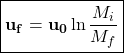Engineers use to speak about the so-called mass ratio, although sometimes the reciprocal definition is also used for such a ratio so be aware, and in terms of this the Tsiokolski’s equation reads: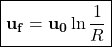We can invert this equation as well, in order to getExample: Calculate the fraction of mass of a one-stage rocket to reach the Earth’s orbit. Typical values forandshow that the mass ratio is equal to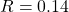. Then, only the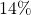of the initial mass reaches the orbit, and the remaining mass is fuel.

Multistage rockets offer a good example of how engineer minds work. They have discovered that a multistage rocket is more effective than the one-stage rocket in terms of maximum attainable speed and mass ratios. The final n-stage launch system for rocketry states that the final velocity is the sum of the different gains in the velocity after the n-th stage, so we can obtain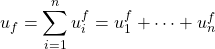After the n-th step, the change in velocity readswhere the i-th mass ratios are defined recursively as the final mass in the n-th step and the initial mass in that step, so we have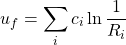and we define the total mass ratio: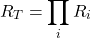If the average effective rocket exhaust velocity is the same in every step/stage, e.g., we get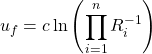or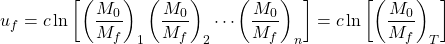The influence of the number of steps, for a given exhaust velocity, in the final attainable velocity can be observed in the next plots:RELATIVISTIC ROCKETS

We proceed now to the relativistic generalization of the previous rocketry. An observer in the laboratory frame observes that total momentum is conserved, of course, and so:whereis the velocity increase in the rocket with a rest mass M’ in the instantaneous reference frame of the moving rocket S’. It is NOT equal to its velocity increase measured in the unprimed reference frame, du. Due to the addition theorem of velocities in SR, we havewhere u is the instanteneous velocity of the rocket with respect to the laboratory frame S. We can perform a Taylor expansion of the denominator in the last equation, in order to obtain: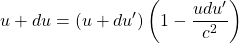and thenand finally, we get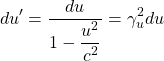Plugging this equation into the above equation for mass (momentum), and integrating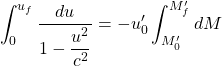we deduce that the relativistic version of the Tsiolkovski’s rocket equation, the so-called relativistic rocket equation, can be written as:We can suppress the primes if we remember that every data is in the S’-frame (instantaneously), and rewrite the whole equation in the more familiar way: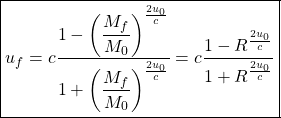where the mass ratio is defined as before. Now, comparing the above equation with the rapidity/maximum velocity in the uniformly accelerated motion: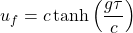we get that relativistic rocket equation can be also written in the next manner: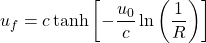or equivalently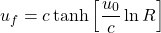since we have in this case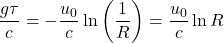and thus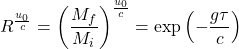If the propellant particles move at speed of light, e.g., they are “photons” or ultra-relativistic particles that move close to the speed of light we have the celebrated “photon rocket”. In that case, setting, we would obtain that: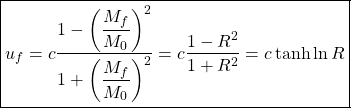and where for the photon rocket (or the ultra-relativistic rocket) we have as wellFinal remark: Instead of the mass ratio, sometimes is more useful to study the ratio fuel mass/payload. In that case, we set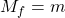and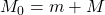, where M is the fuel mass and m is the payload. So, we would writeso then the ratio fuel mass/payload will be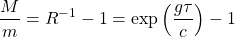We are ready to study the interstellar trip with our current knowledge of Special Relativity and Rocketry. We will study the problem in the next and final post of this fascinating thread. Stay tuned!

This site uses Akismet to reduce spam. Learn how your comment data is processed.